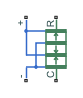# Piezo Stack

Electrical and force characteristics of piezoelectric stacked actuator

•Libraries:
Simscape / Electrical / Electromechanical / Mechatronic Actuators

## Description

The Piezo Stack block represents the electrical and force characteristics of a piezoelectric stacked actuator using the following equations:

`$\begin{array}{l}S={s}^{E}T+{d}^{\text{'}}E\\ D=dT+{\epsilon }^{T}E\end{array}$`

where

• S is the strain tensor.

• T is the stress tensor.

• E is the electric field vector.

• D is the electric displacement vector.

• sE is the elastic compliance matrix when subjected to a constant electric field.

• d is the piezoelectric constant matrix.

• εT is the permittivity measured at a constant stress.

Note

The block models one-dimensional lumped parameter behavior, so S, T, E and D are all scalar values.

You can specify the block parameters that determine static force using either datasheet parameters or material properties, as determined by the value of the Parameterization parameter on the Static Force tab of the block dialog box.

The Dynamic Forces tab of the block dialog box lets you include optional effective mass and mechanical damping effects.

• If you specify a nonzero value for the Effective mass parameter or a finite value for the Resonant frequency at constant field parameter, the block attaches a lumped mass to the mechanical R port. When you specify a finite resonant frequency, the block calculates the effective mass to achieve the correct resonant frequency.

• If you specify a nonzero value for the Damping parameter or a finite value for the Mechanical quality factor parameter, the block adds a damping term across the R to C mechanical ports. When you specify a mechanical quality factor, Qm, the block calculates the damping from this parameter value as$\sqrt{Mk}/{Q}_{m}$, where k is the short-circuit device stiffness, or equivalently the stiffness at constant field.

A positive voltage across the electrical + to - ports creates a positive displacement acting from the mechanical C to R ports.

### Variables

To set the priority and initial target values for the block variables before simulation, use the Initial Targets section in the block dialog box or Property Inspector. For more information, see Set Priority and Initial Target for Block Variables.

Nominal values provide a way to specify the expected magnitude of a variable in a model. Using system scaling based on nominal values increases the simulation robustness. You can specify nominal values using different sources, including the Nominal Values section in the block dialog box or Property Inspector. For more information, see System Scaling by Nominal Values.

## Assumptions and Limitations

• The model does not include hysteresis effects.

## Ports

### Conserving

expand all

Electrical conserving port associated with the piezo stack positive terminal.

Electrical conserving port associated with the piezo stack negative terminal.

Mechanical translational conserving port associated with the case.

Mechanical translational conserving port associated with the rotor.

## Parameters

expand all

### Static Force

Select one of the following methods for static force parameterization:

• `Specify from a datasheet` — Provide datasheet parameters that the block converts to static force values.

• `Specify material properties` — Provide material properties that the block converts to static force values.

Cross-sectional area of the stack.

Stack length when no load and no electrical potential are applied.

#### Dependencies

To enable this parameter, set Parameterization to ```Specify from a datasheet```.

Unconstrained displacement of the stack when a voltage of V0 volts is applied.

#### Dependencies

To enable this parameter, set Parameterization to ```Specify from a datasheet```.

Force the stack produces when a voltage of V0 volts is applied and the stack is physically prevented from expanding.

#### Dependencies

To enable this parameter, set Parameterization to ```Specify from a datasheet```.

Voltage used to determine the no-load displacement and blocking force.

#### Dependencies

To enable this parameter, set Parameterization to ```Specify from a datasheet```.

Capacitance.

#### Dependencies

To enable this parameter, set Parameterization to ```Specify from a datasheet```.

Thickness of each layer in the piezo stack.

#### Dependencies

To enable this parameter, set Parameterization to ```Specify material properties```.

Number of layers in the piezo stack.

#### Dependencies

To enable this parameter, set Parameterization to ```Specify material properties```.

Mechanical strain per unit electric field applied.

#### Dependencies

To enable this parameter, set Parameterization to ```Specify material properties```.

Permittivity or dielectric displacement per unit electric field measured at constant stress.

#### Dependencies

To enable this parameter, set Parameterization to ```Specify material properties```.

Strain produced in a piezoelectric material per unit of stress applied.

#### Dependencies

To enable this parameter, set Parameterization to ```Specify material properties```.

### Dynamic Forces

Select one of the following methods for dynamic force parameterization:

• `Specify from a datasheet` — Provide datasheet parameters that the block converts to dynamic force values.

• `Specify material properties` — Provide material properties that the block converts to dynamic force values.

Frequency at which the actuator naturally resonates if mechanically perturbed with the electrical ports shorted.

#### Dependencies

To enable this parameter, set Parameterization to ```Specify from a datasheet```.

Factor that affects the damping across the R and C mechanical ports.

#### Dependencies

To enable this parameter, set Parameterization to ```Specify from a datasheet```.

Translational damping term.

#### Dependencies

To enable this parameter, set Parameterization to ```Specify from a datasheet```.

Mass that approximates the distributed dynamics of the device and causes the stack to resonate at the correct frequency when attached to the mechanical R port. This mass is usually about one third of the actual stack mass.

#### Dependencies

To enable this parameter, set Parameterization to ```Specify from a datasheet```.

## Version History

Introduced in R2008b